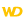HelpWLanguageWLanguage functionsControls, pages and windowsDrawing functionsPrefix syntaxDrawing functions on picLayer variablesDrawArcPresentationExampleCoordinates of the arcDrawing a solid figureDrawing in PHPDrawing in Browser codeRelated examplesSee alsoAlphaBlendBackgroundBlurChangeModeDrawArcDrawChordDrawCircleDrawLineDrawPointDrawPolygonDrawPolylineDrawRectangleDrawRectangleGradientDrawRoundedRectangleDrawSliceDrawTextDrawTextRTFEndDrawingFillFontHorizontalSymmetryInvertColorPenPixelColorPixelOpacityResizeRotationStartDrawingVerticalSymmetry
• Coordinates of the arc
• Drawing a solid figure
• Drawing in PHP
• Drawing in Browser code
WINDEVWEBDEVWINDEV MobileOthers<picLayer variable>.DrawArc (Function)
In french: <Variable picCalque>.DessineArc
Draws a circle arc or an ellipse arc in a variable of type picLayer. The arc is drawn counterclockwise.
Example
MyImage is WDPic = "Test.gif"
MyLayer is picLayer

// Draw a light yellow arc of circle on the layer
MyLayer.DrawArc(10, 10, 50, 50, 20, 20, 40, 50, LightYellow)

IMG_MyDrawing = MyImage
Syntax

Drawing an arc

<picLayer image>.DrawArc(<X1> , <Y1> , <X2> , <Y2> , <X3> , <Y3> , <X4> , <Y4> [, <Line color> [, <Line thickness>]])
<picLayer image>: picLayer variable
Name of the picLayer variable to be used.
<X1>: Integer
X-coordinate (in pixels) of the upper-left corner of the rectangle containing the circle.
<Y1>: Integer
Y-coordinate (in pixels) of the upper-left corner of the rectangle containing the circle.
<X2>: Integer
X-coordinate (in pixels) of the lower-right corner of the rectangle containing the circle.
<Y2>: Integer
Y-coordinate (in pixels) of the lower-right corner of the rectangle containing the circle.
<X3>: Integer
X-coordinate (in pixels) of the starting point of the arc.
<Y3>: Integer
Y-coordinate (in pixels) of the starting point of the arc.
<X4>: Integer
X-coordinate (in pixels) of the end point of the arc.
<Y4>: Integer
Y-coordinate (in pixels) of the end point of the arc.
<Line color>: Integer or constant (optional)
Color of the arc of circle. This color can correspond to:
• an RGB color (returned by RGB),
• an HSL color (returned by HSL),
• a WLanguage preset color,
•a variable of type Color.
If this parameter is not specified, the line color:
• is Transparent if <Image>.Pen has not been used beforehand,
• corresponds to the color specified in the last call to <Image>.Pen.
<Line thickness>: Optional integerThickness (in pixels) of the arc of circle to draw. If this parameter is not specified, the value taken into account can be:
Remarks

Coordinates of the arc

If the start and end points are not located on the border of the circle (or on the border of the ellipse), the point taken into account corresponds to the intersection between the circle and the line that joins the specified point and the center of the rectangle (which means the center of the circle).The origin used to calculate the coordinates corresponds to the upper-left corner of the image (coordinates: (0,0)).

Drawing a solid figure

To draw a solid figure (a full arc), use <picLayer variable>.DrawChord or <picLayer variable>.DrawSlice.
Related Examples:Unit examples (WINDEV): The drawing functions [ + ] Using the main drawing functions of WINDEV to:- Initialize an Image control for drawing- Draw simple shapes- Write a text into a drawing- Change the color in a drawing
Business / UI classification: Neutral code
Component: wd280pnt.dll
Minimum version required
• Version 25